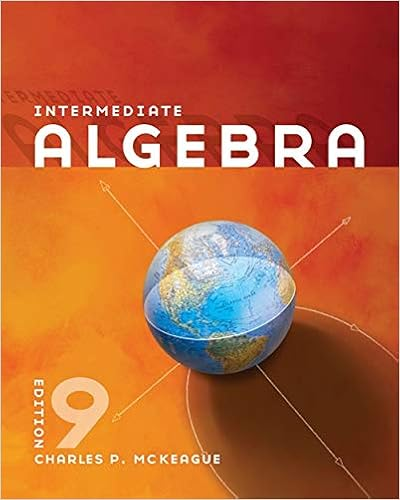assignment sheet-WA2_MAT-115-mar18.docx - MAT-115...

• Homework Help
• 10
• 86% (14) 12 out of 14 people found this document helpful

This preview shows page 1 - 4 out of 10 pages.

We have textbook solutions for you!
The document you are viewing contains questions related to this textbook.The document you are viewing contains questions related to this textbook.
Chapter 4 / Exercise 7
Intermediate Algebra
MckeagueExpert Verified
MAT-115: INTERMEDIATE ALGEBRA Written Assignment 2 2.1: Use a General Strategy to Solve Linear Equations S olve the linear equation. 1. 3 ( x + 4 )= 24 3 ( x + 4 ) 3 = 24 3 X+4= 24 3 X+4=8 X=-4+8 X=4 2. 5 ( 2 n + 3 )= 25 5 ( 2 n + 3 ) 5 = 25 5 ¿ ¿ 2n+3)= 25 5 −(2 n )−1⋅3= 25 5 -2-3=5 -2n=8 - n 1 = 8 2 −(−1 n )= 8 2 n=-4 C lassify each equation as a conditional equation, an identity, or a contradiction. T hen, find the solution. 3. 2 ( 6 x 1 )= 3 ( 4 x + 8 ) contradiction Copyright © 2018 by Thomas Edison State University. All rights reserved.
We have textbook solutions for you!
The document you are viewing contains questions related to this textbook.The document you are viewing contains questions related to this textbook.
Chapter 4 / Exercise 7
Intermediate Algebra
MckeagueExpert Verified
2(6 x )+2⋅−1=3(4 x +8) 12 x −2=3(4 x +8) 12 x −2=3(4 x )+3⋅8 12 x −2=12 x +24 12 x −2−12 x =24 24=−2 No solution 4. 8 ( 3 x + 2 )= 28 x + 16 4 x 28 x +16−4 x =8(3 x +2) 24 x +16=8(3 x +2) 24 x +16=8(3 x )+8⋅2 24 x +16=24 x +16 24 x +16−24 x =16 16=16 Solve. 5. 3 4 x + 1 2 = 1 4 6. x + 1 2 = 3 4 , 1 4 X= 3 4 , 1 2 , 1 4 7. 0.8 x + 0.7 = 0.6 x 3.3 0.8 x +0.7−0.6 x =−3.3 0.2 x +0.7=−3.3 0.2 x =−0.7−3.3 0.2 x =−4 0.2 x 0.2 = 4 0.2 X= 4 0.2 X=-20 Copyright © 2018 by Thomas Edison State University. All rights reserved.
2.2: Use a Problem Solving Strategy Solve using a problem solving strategy. 8. Three-fifths of the people at a concert are children. If there are a total of 60 people at the concert, what is the total number of children at the concert? What is the total number of adults at the concert?
•••